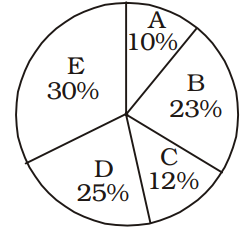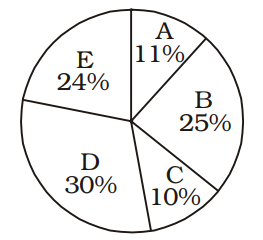# IBPS Clerk Numerical Ability Online Test 3 Practice Questions

1.

In a class of 30 students and 2 teachers, each student got sweets that are 20% of the total number of students and each teacher got sweets that are 30% of the total number of students. How many sweets were there ?

a

178

b

180

c

208

d

188

e

None of these

2.

What is the compound interest accrued on an amount of Rs. 12000 at the rate of 9 p.c.p.a. at the end of 2 years.

a

Rs. 2986

b

Rs. 2545

c

Rs. 2775.4

d

Rs. 2257.2

e

None of these

3.

If (58)2 is added to the square of a number, the answer so obtained is 5668. What is the number ?

a

56

b

47

c

33

d

51

e

None of these

4.

What approximate value should come in place of the question mark (?) in the following question ? 45.709 ÷ 11.342 × 6.667 = ?

a

56

b

88

c

112

d

46

e

27

5.

The owner of a Television shop charges his customer 16% more than the cost price. If a customer paid Rs. 16588 for a Television, then what was the cost price of the Television ?

a

Rs. 12000

b

Rs. 15500

c

Rs. 14300

d

Rs. 13800

e

None of these

6.

Which number should replace both the question marks (?) in the following equation ?

$frac{?}{188}=frac{47}{?}$

a

66

b

88

c

124

d

94

e

None of these

7.

Ms. Anisha deposits an amount of Rs. 35000 to obtain a simple interest at the rate of 15 p.c.p.a. for 4 years. What total amount will Ms. Anisha get at the end of 4 years ?

a

Rs. 60500

b

Rs. 56000

c

Rs. 48500

d

Rs. 52000

e

None of these

8.

A single person takes 8 minutes to type a page. If from 1.00 p.m. to 2.00 p.m., 1710 pages are to be typed, how many persons should be employed on this job ?

a

256

b

221

c

249

d

207

e

None of these

9.

In an examination it is required to get 380 of the aggregate marks to pass. A student gets 32% of marks and is declared failed by 76 marks. What are the maximum aggregate marks a student can get ?

a

945

b

950

c

975

d

Cannot be determined

e

None of these

10.

The cost of 20 folders and 15 pens is Rs. 995. What is the cost of 12 folders and 9 pens ?

a

Rs. 447

b

Rs. 597

c

Rs. 652

d

Cannot be determined

e

None of these

11.

A sum of money is divided among A, B, C and D in the ratio of 4 : 6 : 11 : 13 respectively. If the share of C is Rs. 7854, then what is the total amount of money of B and D together ?

a

Rs. 12,784

b

Rs. 15,676

c

Rs. 13,566

d

Rs. 16,928

e

None of these

12.

One-seventh of a number is 51. What will be 64% of that number ?

a

228.48

b

238.24

c

248.12

d

198.36

e

None of these

13.

The average age of a man and his son is 48 years. The ratio of their ages is 11 : 5 respectively. What will be ratio of their ages after 6 years ?

a

5 : 3

b

4 : 3

c

6 : 5

d

2 : 1

e

None of these

14.

If an amount of Rs. 97836 is distributed equally amongst 31 children, how much amount would each child get ?

a

Rs. 3156

b

Rs. 3756

c

Rs. 3356

d

Rs. 3556

e

None of these

15.

Of the two numbers, 48 per cent of first number is 60 per cent of the second number. What is the respective ratio of the first number to the second number ?

a

5 : 4

b

4 : 7

c

3 : 4

d

Cannot be determined

e

None of these

16.
Directions(Q.16 to Q.20 ):

What will come in place of question mark (?) in the given number series?

5 9 25 89 ? 1369

a

349

b

341

c

355

d

343

e

345

17.

61 62 54 81 17 ?

a

145

b

144

c

142

d

138

e

140

18.

6 11 18 29 46 ?

a

69

b

60

c

75

d

71

e

79

19.

6 17 50 149 ? 1337

a

454

b

442

c

446

d

452

e

432

20.

26 13 13 19.5 ? 97.5

a

45

b

48

c

41

d

39

e

35

21.
Directions(Q.21 to Q.30 ):

What should come in place of the question mark (?) in the following questions ?

? ÷ 40 × 9 = 378

a

1648

b

1616

c

1680

d

1696

e

None of these

22.

$2 frac{1}{4}+3 frac{3}{4}-1 frac{2}{3}=?$

a

$4 frac{3}{5}$

b

$4 frac{1}{4}$

c

$4 frac{1}{3}$

d

$4 frac{2}{3}$

e

None of these

23.

2637 ÷ 36 = ?

a

68

b

73.25

c

66.5

d

71

e

None of these

24.

66% of 546 – 43% of 439 = ?

a

111.71

b

171.59

c

103.57

d

138.63

e

None of these

25.

18 × 8 + (?)2 = (15)2

a

27

b

9

c

18

d

81

e

None of these

26.

546 + 222 ÷ 6 × 9 = ?

a

982

b

997

c

839

d

879

e

None of these

27.

$5 frac{1}{8}$ of $208+786=2000-?$

a

112

b

124

c

184

d

148

e

None of these

28.

(62)2 + (14)2 = (?)2 + 559

a

59

b

53

c

48

d

56

e

None of these

29.

11.6 × 8.9 × 5.1 = ?

a

398.264

b

526.524

c

664.358

d

468.428

e

None of these

30.

1354 + 1184 = ? % of 5640

a

52

b

42

c

36

d

45

e

None of these

31.
Directions(Q.31 to Q.35 ):

Refer to the pie charts and answer the given questions.

The following data are regarding number of students (boys + girls) studying in standard Xth of various schools (A, B, C, D and E in the year 2010)

Total number of students (Boys + Girls) = 3000Total number of boys = 1600The number of girls studying in school C, is what per cent of total number of students (boys + girls) studying in the same school?

a

50

b

$55 frac{5}{9}$

c

$60 frac{1}{3}$

d

48

e

$42 frac{5}{8}$

32.

What is the difference between the total number of students (boys + girls) in schools A and B together and the total number of students (boys + girls) in schools D and E together?

a

600

b

640

c

680

d

620

e

660

33.

What is the average number of boys studying in schools A and B ?

a

248

b

272

c

288

d

280

e

278

34.

The number of girls studying in school E is approximately what per cent more than the number of boys studying in the same school ?

a

45

b

50

c

40

d

30

e

34

35.

What is the respective ratio between the number of boys and the number of girls studying in school D ?

a

18 : 7

b

16 : 7

c

12 : 7

d

16 : 9

e

14 : 9

Rate This:
NaN / 5 - 1 votes

### IBPS Clerk Numerical Ability Online Test 3 Practice Questions

» Your comments will be displayed only after manual approval.